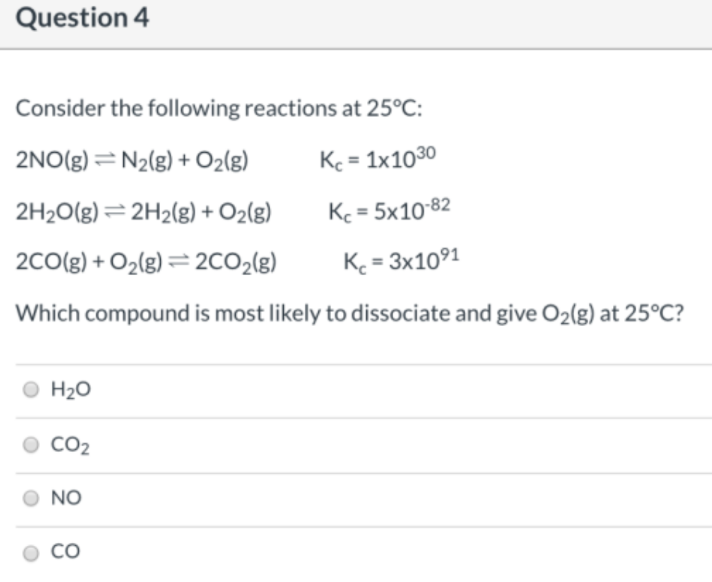# Consider the following reactions at 25 °C: 2NO(g) ⇌ N2(g) + O2(g) Kc = 1x10^30 2H2O(g) ⇌ 2H2(g) + O2(g) Kc = 5x10^-82 2CO(g) + O2(g) ⇌ 2CO2(g) Kc = 3x10^91 Which compound is most likely to dissociate and give O2(g) at 25 °C? a) H2O b) CO2 c) NO d) CO